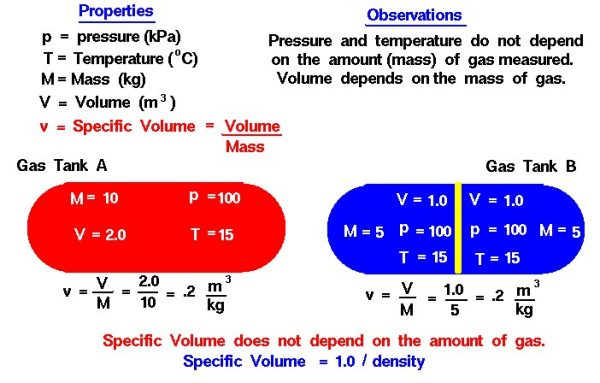# Specific Volume## Gas State

The state of a gas is defined by various properties which we can observe with our senses, including the gas pressure (p), temperature (T), mass (number of moles – m), and volume (V) which contains the gas. It is observed that, if we have a certain amount (mass or volume) of gas present, the value of the temperature and pressure does not depend on the amount of gas that we examine. For example, suppose we have a tank of gas. If we insert a plate into the tank which cuts the volume in half, the temperature in each half remains the same, as does the pressure.

The value of pressure and temperature does not depend on the amount of gas used in the measurement. The mass of the gas, on the other hand, does depend on the volume. Cutting the volume in two cuts the mass in two. The mass in each section of the tank is one-half the mass of the entire tank. The mass depends on the volume and, in turn, the volume depends on the mass. If we maintain the pressure and temperature of this gas and fill an object which can vary its volumes, like a balloon, or a cylinder with a sliding end, the final volume depends directly on the amount of the gas that we inject.

Properties that depend on the amount of gas are called extensive properties, while properties that do not depend on the amount of gas are called intensive properties. When performing a thermodynamic analysis, it is much easier to deal with only intensive properties since we can eliminate the mass from the analysis. Since the mass and volume are directly related to each other under static conditions, we can define a new property called the specific volume which is equal to the volume divided by the mass. Specific volume is an intensive property of the gas, as shown in our example. The specific volume of the original tank is the same as the specific volume in each half. The “specific” of specific volume simply means “divided by mass”.

A closer examination of the definition for specific volume shows that the specific volume v is the inverse of the gas density rho ($$\bf\rho$$):

$$\LARGE v=\frac{1}{\rho}$$

Either the specific volume or the density can be used in defining the state of the gas using only intensive variables. For many fluid dynamic (moving) applications, the mass varies from one location to another, and aerodynamicists normally use the density as the intensive property.

Provide feedback